Start typing, then use the up and down arrows to select an option from the list.# The Colligative Properties Example 2

Jules Bruno
180views
1
which of the following compounds will have the largest value for the Van Hoff factor? Alright, So for the first one, we have aluminum chloride, which is composed, which is an ionic compound, so it breaks up into ions. It's composed of one aluminum ion and three chloride ions, So in total, that's four ions. So I equals four for the next one. It's Covalin because only non metals together, So I equals one for the next one. It's ironic again, because zinc is a metal. Oxygen is a nonmetal, so it breaks up into zinc ion and oxide ion. That's two ions total, so I equals two. Next we have ammonia, which is NH three. It's Kovalev because only non metals. So I equals one. And then we have P two s five, which is also Covalin. It's only non metals together, so I equals one. We can see the one with the greatest Van Hoff factor. Value is a I equals four for aluminum chloride. So here the answer would be option a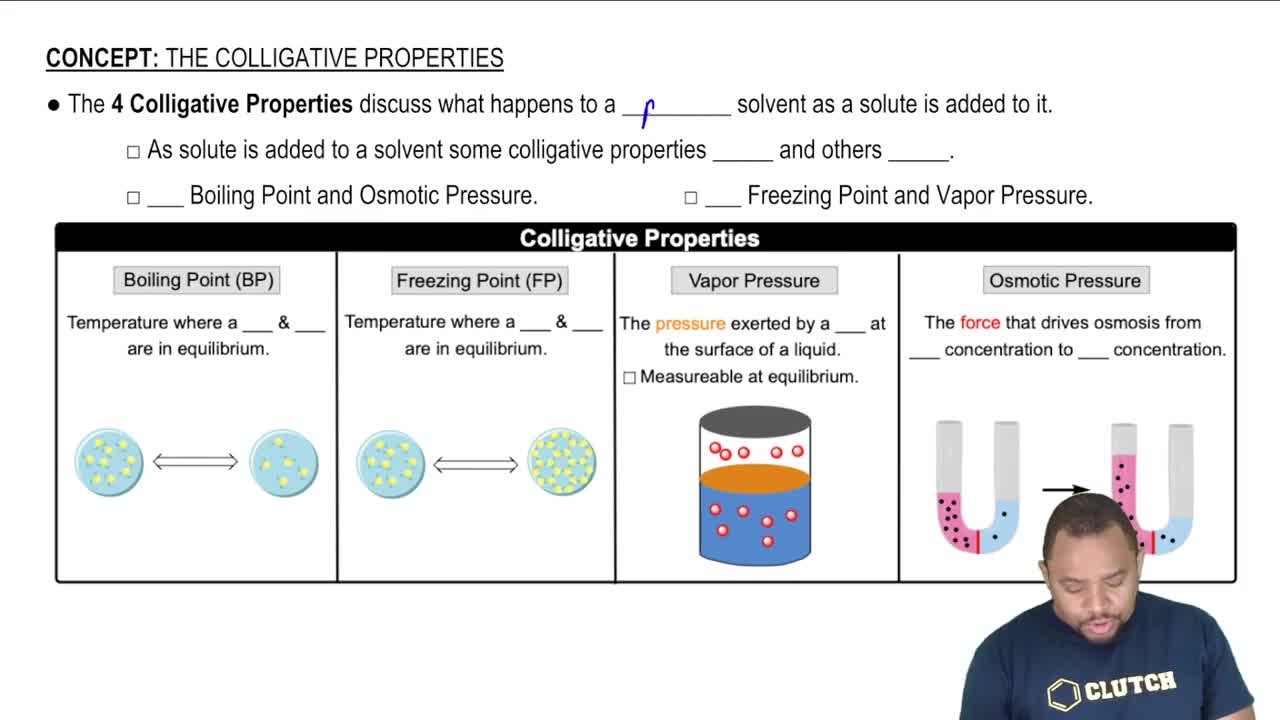02:43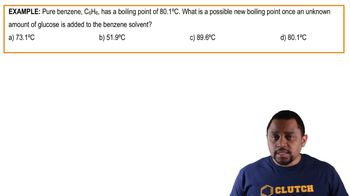00:50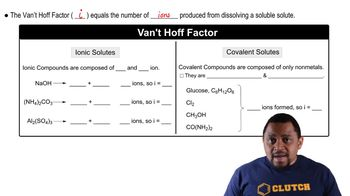02:50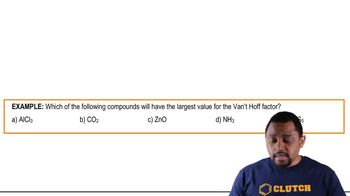01:05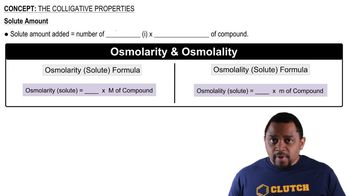01:26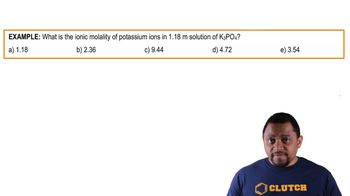01:03